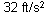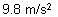﻿ Material Variables
Manufacturing Solutions

# Material Variables# Material VariablesThe variables for the material update parameters are:

 E = Young’s Modulus YS = Yield Stress of material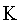= Strength coefficient (power law equation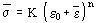)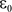= Pre-strain coefficient n = Strain-hardening exponent (measures stretch ability/work hardening of material) r = Plastic strain ratio (measures deep draw ability of material)

Note:   Material units must be consistent for all parameters.

The following equations are commonly used to calculateand: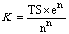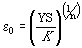Where:

e        =        2.718

TS        =        tensile strength

YS        =        yield stress

Some common unit conversions are listed below:

 USCU – SI SI - USCU Length 1 in = 25.4 mm 1 mm = 0.03937 in. Force 1 lb = 4.448 N 1 N = 0.2248 lb Stress 1 Ksi = 6.895 Mpa 1 Mpa = 0.145 Ksi Mass 1 lbm = 0.4536 kg 1 lb = 0.0005 tons 1 kg = 2.205 lbm 1 ton = 2000 lbs Gravity